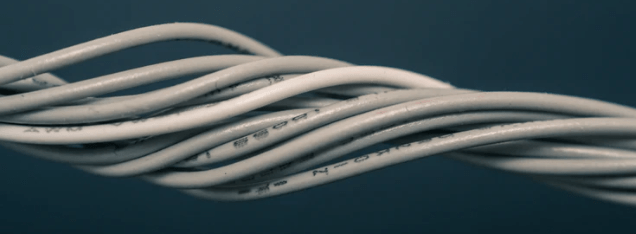# EE01 – 0,4KV SYSTEMS CABLE CROSS SECTION ESTIMATOR

No account yet? Register

Just enter distance and power to get cross sectional area and amperage estimation

JavaScript program to calculate multiplication and division of two numbers
Distance: m

Power: kW

Minimum cross section is (in mm^2):

Current calculated is (in A):

NOTE: THESE ARE THE ROUGHLY CALCULATED VALUES AND MINIMUMAdd to favorites